Next: Chain Graphs Up: Introduction Previous: KMC and DPS Averages   Contents

## Mean Escape Times

Since the mean first passage time between states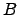and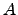, or the escape time from a subgraph, is of particular interest, we first illustrate a means to derive formulae for these quantities in terms of pathway probabilities.

The average time taken to traverse a path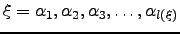is calculated as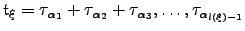, where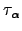is the mean waiting time for escape from node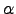, as above,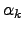identifies the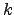th node along path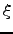, and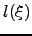is the length of path.The mean escape time from a graph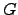if started from node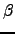is then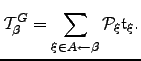(6.20)

If we multiply every branching probability,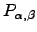, that appears in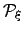by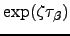then the mean escape time can be obtained as: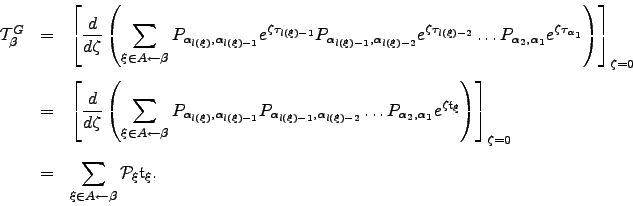(6.21)

This approach is useful if we have analytic results for the total probability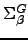, which may then be manipulated into formulae for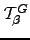, and is standard practice in probability theory literature [208,209]. The quantity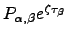is similar to the `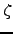probability' described in Reference GoldhirschG86. Analogous techniques are usually employed to obtainand higher moments of the first-passage time distribution from analytic expressions for the first-passage probability generating function (see, for example, References Raykin92,MurthyK89). We now define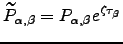and the related quantities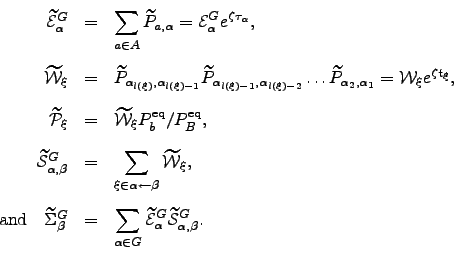(6.22)

Note that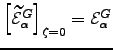etc., while the mean escape time can now be written as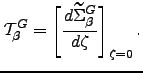(6.23)

In the remaining sections we show how to calculate the pathway probabilities,, exactly, along with pathway averages, such as the waiting time. Chain graphs are treated in Section 4.2 and the results are generalised to arbitrary graphs in Section 4.3.Next: Chain Graphs Up: Introduction Previous: KMC and DPS Averages   Contents
Semen A Trygubenko 2006-04-10